# 9 Introduction to the Rrms Package: The Linear Model

Some of the purposes of the rms package are to

A

• make everyday statistical modeling easier to do
• make modern statistical methods easy to incorporate into everyday work
• make it easy to use the bootstrap to validate models
• provide “model presentation graphics”

## 9.1 Formula Language and Fitting Function

• Statistical formula in R:
B
y ~ x1 + x2 + x3

y is modeled as $$\alpha + \beta_{1}x_{1} + \beta_{2}x_{2} + \beta_{3} x_{3}$$

• y is the dependent variable/response/outcome, x’s are predictors (independent variables)
• The formula is the first argument to a fitting function (just as it is the first argument to a trellis graphics function)
• rms (regression modeling strategies) package makes many aspects of regression modeling and graphical display of model results easier to do
• rms does a lot of bookkeeping to remember details about the design matrix for the model and to use these details in making automatic hypothesis tests, estimates, and plots. The design matrix is the matrix of independent variables after coding them numerically and adding nonlinear and product terms if needed.
• rms package fitting function for ordinary least squares regression (what is often called the linear model or multiple linear regression): ols
• Example:
C
f <- ols(y ~ age + sys.bp, data=mydata)
• age and sys.bp are the two predictors (independent variables) assumed to have linear and additive effects (do not interact or have synergism)
• mydata is an R data frame containing at least three columns for the model’s variables
• f (the fit object) is an R list object, containing coefficients, variances, and many other quantities
• Below, the fit object will be f throughout. In practice, use any legal R name, e.g. fit.full.model

## 9.2 Operating on the Fit Object

D
• Regression coefficient estimates may be obtained by any of the methods listed below
f$coefficients f$coef          # abbreviation
coef(f)         # use the coef extractor function
coef(f)      # get intercept
f$coef # get 2nd coefficient (1st slope) f$coef['age']   # get coefficient of age
coef(f)['age']  # ditto
• But often we use methods which do something more interesting with the model fit.
• print(f): print coefficients, standard errors, $$t$$-test, other statistics; can also just type f to print
• fitted(f): compute $$\hat{y}$$
• predict(f, newdata): get predicted values, for subjects described in data frame newdata1
• r <- resid(f): compute the vector of $$n$$ residuals (here, store it in r)
• formula(f): print the regression formula fitted
• anova(f): print ANOVA table for all total and partial effects
• summary(f): print estimates partial effects using meaningful changes in predictors
• Predict(f): compute predicted values varying a few predictors at a time (convenient for plotting)
• ggplot(p): plot partial effects, with predictor ranging over the $$x$$-axis, where p is the result of Predict
• g <- Function(f): create an R function that evaluates the analytic form of the fitted function
• nomogram(f): draw a nomogram of the model

Note: Some of othe functions can output html if options(prType='html') is set, as is done in this chapter. When using that facility you often need to add results='asis' in the knitr chunk header.

## 9.3 The rmsdatadist Function

E

To use Predict, summary, or nomogram in the rms package, you need to let rms first compute summaries of the distributional characteristics of the predictors:

dd <- datadist(x1,x2,x3,...)   # generic form
dd <- datadist(age, sys.bp, sex)
dd <- datadist(mydataframe)    # for a whole data frame
options(datadist='dd')         # let rms know where to find

Note that the name dd can be any name you choose as long as you use the same name in quotes to options that you specify (unquoted) to the left of <- datadist(...). It is best to invoke datadist early in your program before fitting any models. That way the datadist information is stored in the fit object so the model is self-contained. That allows you to make plots in later sessions without worrying about datadist. datadist must be re-run if you add a new predictor or recode an old one. You can update it using for example

dd <- datadist(dd, cholesterol, height)
# Adds or replaces cholesterol, height summary stats in dd

## 9.4 Short Example

F

Consider the lead exposure dataset from B. Rosner Fundamentals of Biostatistics and originally from Landrigan PJ et al, Lancet 1:708-715, March 29, 1975. The study was of psychological and neurologic well-being of children who lived near a lead smelting plant. The primary outcome measures are the Wechsler full-scale IQ score (iqf) and the finger-wrist tapping score maxfwt. The dataset is available at hbiostat.org/data and can be automatically downloaded and load()’d into R using the Hmisc package getHdata function. For now we just analyze lead exposure levels in 1972 and 1973, age, and maxfwt2.

G
Note: To easily run all the following commands, open fharrell.com/code/bbr.zip and then open the file 8-rmsintro.r contained in the .zip file using RStudio. Commands listed in previous sections were not actually executed so they are marked with the R comment symbol (#) and can be ignored.

# For an Rmarkdown version of similar analyses see
# https://github.com/harrelfe/rscripts/raw/master/lead-ols.md
require(rms)    # also loads the Hmisc package
getHdata(lead)
# Subset variables just so contents() and describe() output is short
# Override units of measurement to make them legal R expressions
lead <- upData(lead,
keep=c('ld72', 'ld73', 'age', 'maxfwt'),
labels=c(age='Age'),
units=c(age='years', ld72='mg/100*ml', ld73='mg/100*ml'))
Input object size:   53928 bytes;    39 variables    124 observations
Kept variables  ld72,ld73,age,maxfwt
New object size:    14096 bytes;    4 variables 124 observations
H
html(contents(lead))

#### Data frame:lead

124 observations and 4 variables, maximum # NAs:25
NameLabelsUnitsStorageNAs
ageAgeyearsdouble 0
ld72Blood Lead Levels, 1972mg/100*mlinteger 0
ld73Blood Lead Levels, 1973mg/100*mlinteger 0
maxfwtMaximum mean finger-wrist tapping scoreinteger25

html(describe(lead))

lead

4 Variables   124 Observations

age: Age years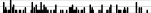nmissingdistinctInfoMeanGmd.05.10.25.50.75.90.95
12407318.9354.074 3.929 4.333 6.167 8.37512.02114.00015.000
 lowest : 3.75 3.83333 3.91667 4 4.16667 highest: 14.25 15 15.25 15.4167 15.9167

ld72: Blood Lead Levels, 1972 mg/100*ml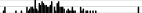nmissingdistinctInfoMeanGmd.05.10.25.50.75.90.95
1240470.99936.1617.2318.0021.0027.0034.0043.0057.0061.85
lowest : 1 2 10 14 18 , highest: 62 64 66 68 99
ld73: Blood Lead Levels, 1973 mg/100*ml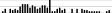nmissingdistinctInfoMeanGmd.05.10.25.50.75.90.95
1240370.99831.7111.0618.1521.0024.0030.5037.0047.0050.85
lowest : 15 16 18 19 20 , highest: 52 53 54 57 58
maxfwt: Maximum mean finger-wrist tapping score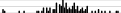nmissingdistinctInfoMeanGmd.05.10.25.50.75.90.95
9925400.99851.9613.833.238.046.052.059.065.072.2
lowest : 13 14 23 26 34 , highest: 74 76 79 83 84
I

dd <- datadist(lead); options(datadist='dd')
dd    # show what datadist computed 
                      age  ld72  ld73 maxfwt
Low:effect       6.166667 27.00 24.00   46.0
Adjust to        8.375000 34.00 30.50   52.0
High:effect     12.020833 43.00 37.00   59.0
Low:prediction   3.929167 18.00 18.15   33.2
High:prediction 15.000000 61.85 50.85   72.2
Low              3.750000  1.00 15.00   13.0
High            15.916667 99.00 58.00   84.0
# Fit an ordinary linear regression model with 3 predictors assumed linear
f <- ols(maxfwt ~ age + ld72 + ld73, data=lead)
J
f         # same as print(f)

Linear Regression Model

 ols(formula = maxfwt ~ age + ld72 + ld73, data = lead)

Frequencies of Missing Values Due to Each Variable
 maxfwt    age   ld72   ld73
25      0      0      0

Model Likelihood
Ratio Test
Discrimination
Indexes
Obs 99 LR χ2 62.25 R2 0.467
σ 9.5221 d.f. 3 R2adj 0.450
d.f. 95 Pr(>χ2) 0.0000 g 10.104

Residuals

      Min       1Q   Median       3Q      Max
-33.9958  -4.9214   0.7596   5.1106  33.2590

β S.E. t Pr(>|t|)
Intercept  34.1059  4.8438 7.04 <0.0001
age   2.6078  0.3231 8.07 <0.0001
ld72  -0.0246  0.0782 -0.31 0.7538
ld73  -0.2390  0.1325 -1.80 0.0744
K
coef(f)   # retrieve coefficients
 Intercept        age       ld72       ld73
34.1058551  2.6078450 -0.0245978 -0.2389695 
specs(f, long=TRUE)   # show how parameters are assigned to predictors,
ols(formula = maxfwt ~ age + ld72 + ld73, data = lead)

Units     Label                   Assumption Parameters d.f.
age  years     Age                     asis                  1
ld72 mg/100*ml Blood Lead Levels, 1972 asis                  1
ld73 mg/100*ml Blood Lead Levels, 1973 asis                  1

age  ld72  ld73
Low:effect       6.166667 27.00 24.00
Adjust to        8.375000 34.00 30.50
High:effect     12.020833 43.00 37.00
Low:prediction   3.929167 18.00 18.15
High:prediction 15.000000 61.85 50.85
Low              3.750000  1.00 15.00
High            15.916667 99.00 58.00
                      # and predictor distribution summaries driving plots
L
g <- Function(f)  # create an R function that represents the fitted model
# Note that the default values for g's arguments are medians
g
function (age = 8.375, ld72 = 34, ld73 = 30.5)
{
34.105855 + 2.607845 * age - 0.024597799 * ld72 - 0.23896951 *
ld73
}
<environment: 0x55d32b7af780>
# Estimate mean maxfwt at age 10, .1 quantiles of ld72, ld73 and .9 quantile of ld73
# keeping ld72 at .1 quantile
g(age=10, ld72=21, ld73=c(21, 47))  # more exposure in 1973 decreased y by 6
 54.64939 48.43618
M
# Get the same estimates another way but also get std. errors
predict(f, data.frame(age=10, ld72=21, ld73=c(21, 47)), se.fit=TRUE)
$linear.predictors 1 2 54.64939 48.43618$se.fit
1        2
1.391858 3.140361 

## 9.5 Operating on Residuals

N

Residuals may be summarized and plotted just like any raw data variable.

• To plot residuals vs. each predictor, and to make a q-q plot to check normality of residuals, use these examples:
r <- resid(f)
par(mfrow=c(2,2))   # 2x2 matrix of plots
plot(fitted(f), r); abline(h=0)  # yhat vs. r
with(lead, plot(age,  r));    abline(h=0)
with(lead, plot(ld73, r));    abline(h=0)
qqnorm(r)           # linearity indicates normality
qqline(as.numeric(r))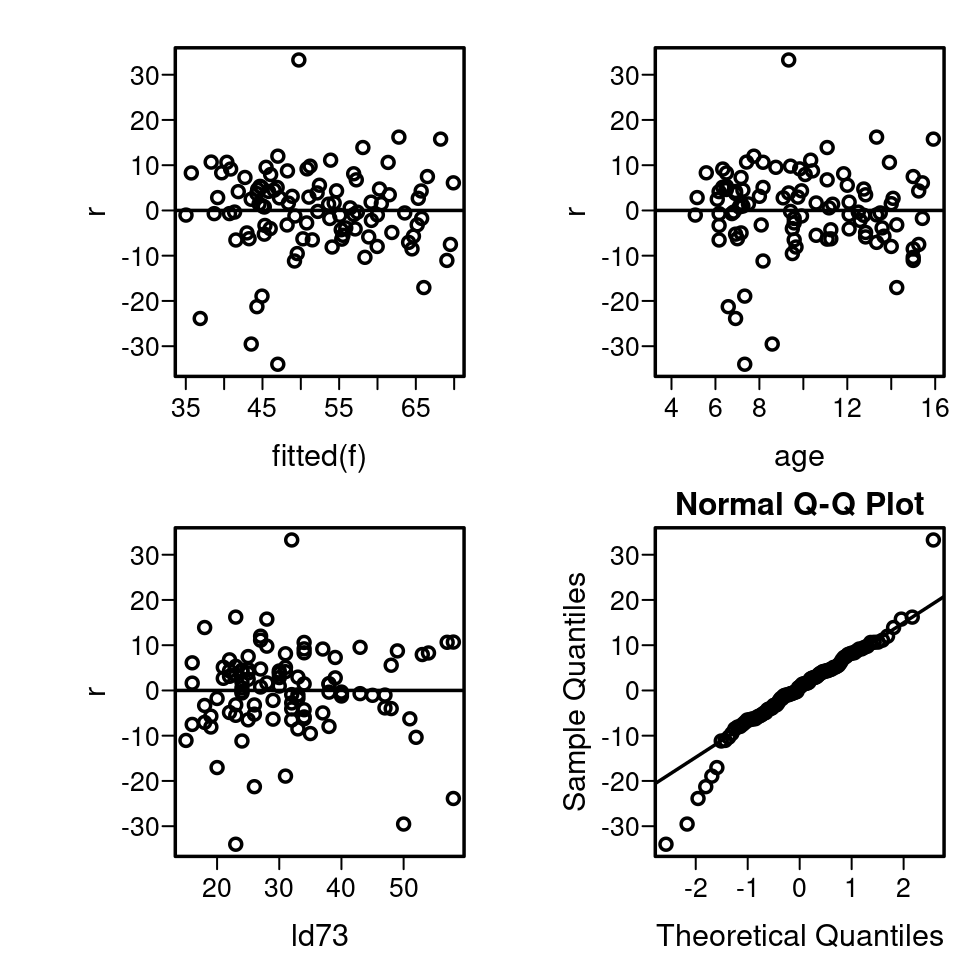## 9.6 Plotting Partial Effects

O

• Predict and ggplot makes one plot for each predictor
• Predictor is on $$x$$-axis, $$\hat{y}$$ on the $$y$$-axis
• Predictors not shown in plot are set to constants
• median for continuous predictors
• mode for categorical ones
• For categorical predictor, estimates are shown only at data values
• 0.95 pointwise confidence limits for $$\hat{E}(y|x)$$ are shown (add conf.int=FALSE to Predict() to suppress CLs)
• Example:
P
ggplot(Predict(f))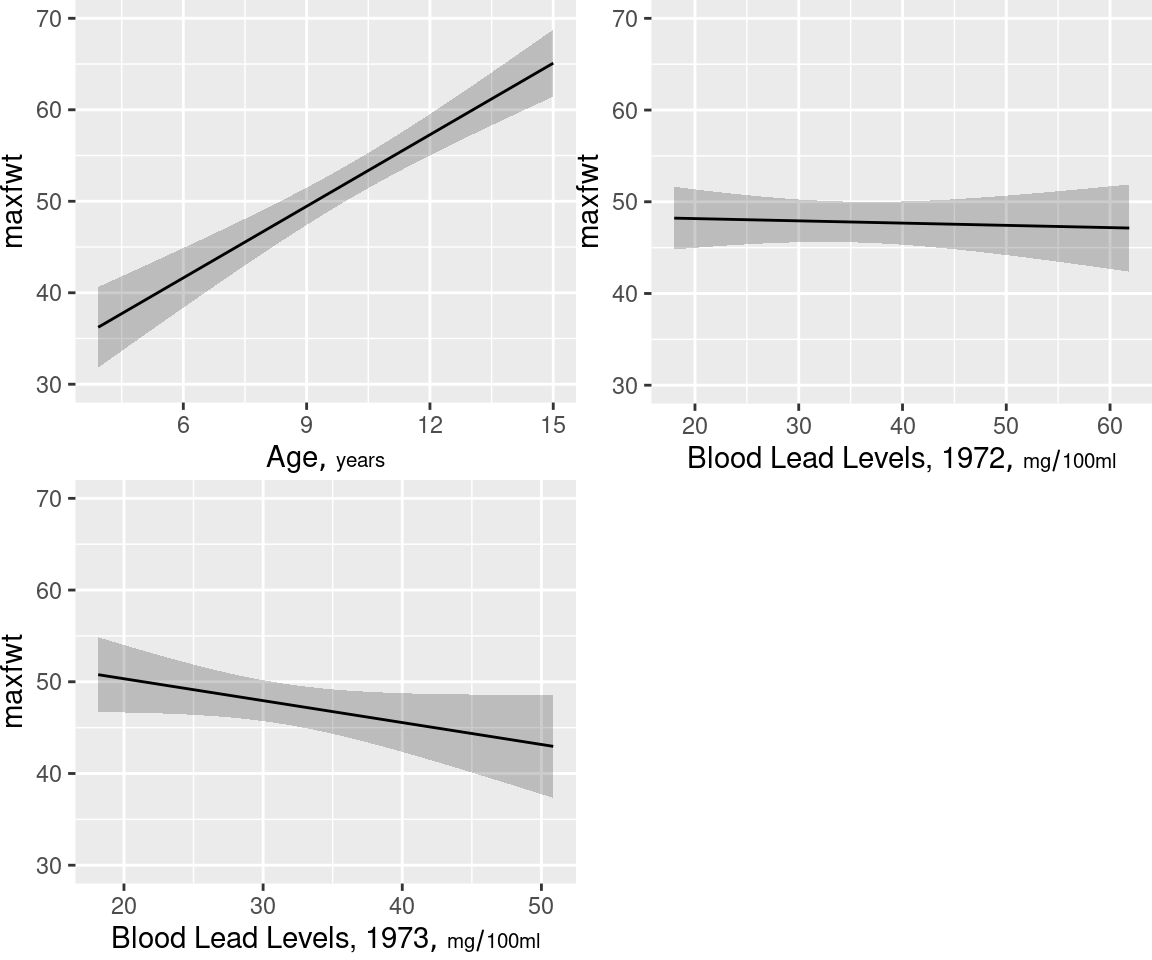• To take control of which predictors are plotted, or to specify customized options:
Q
ggplot(Predict(f, age))   # plot age effect, using default range,
# 10th smallest to 10th largest age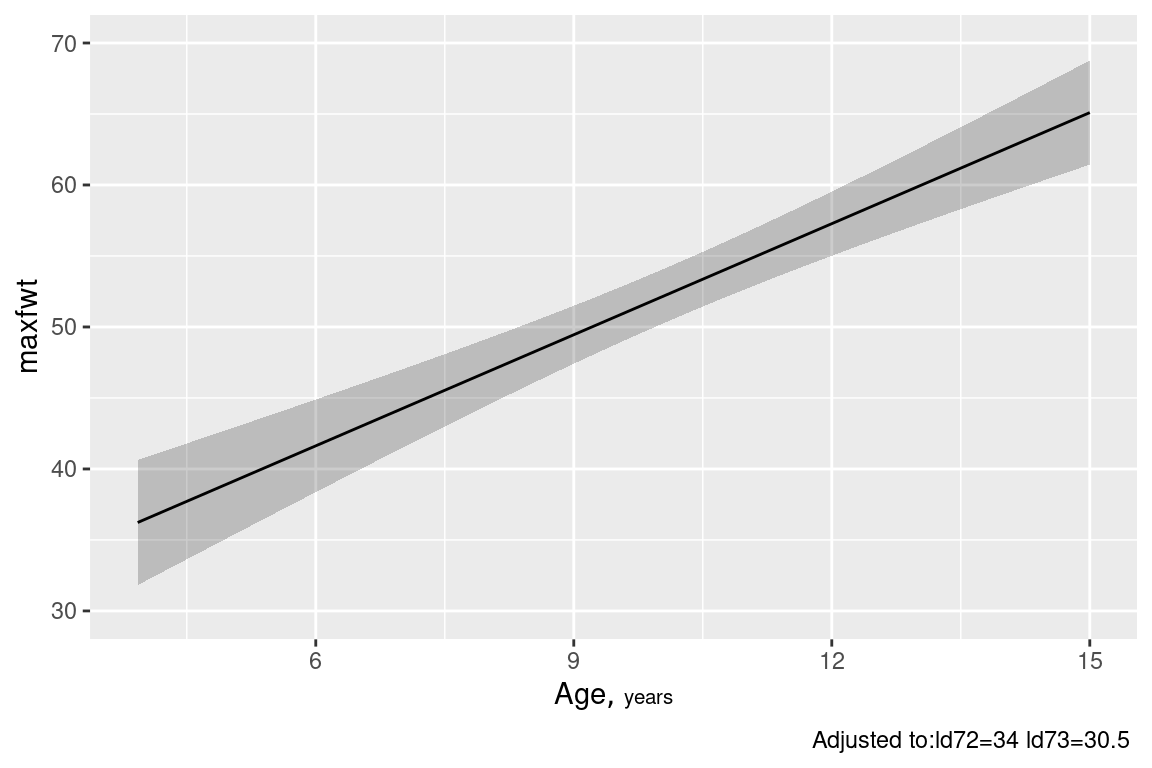R

ggplot(Predict(f, age=3:15))  # plot age=3,4,...,15